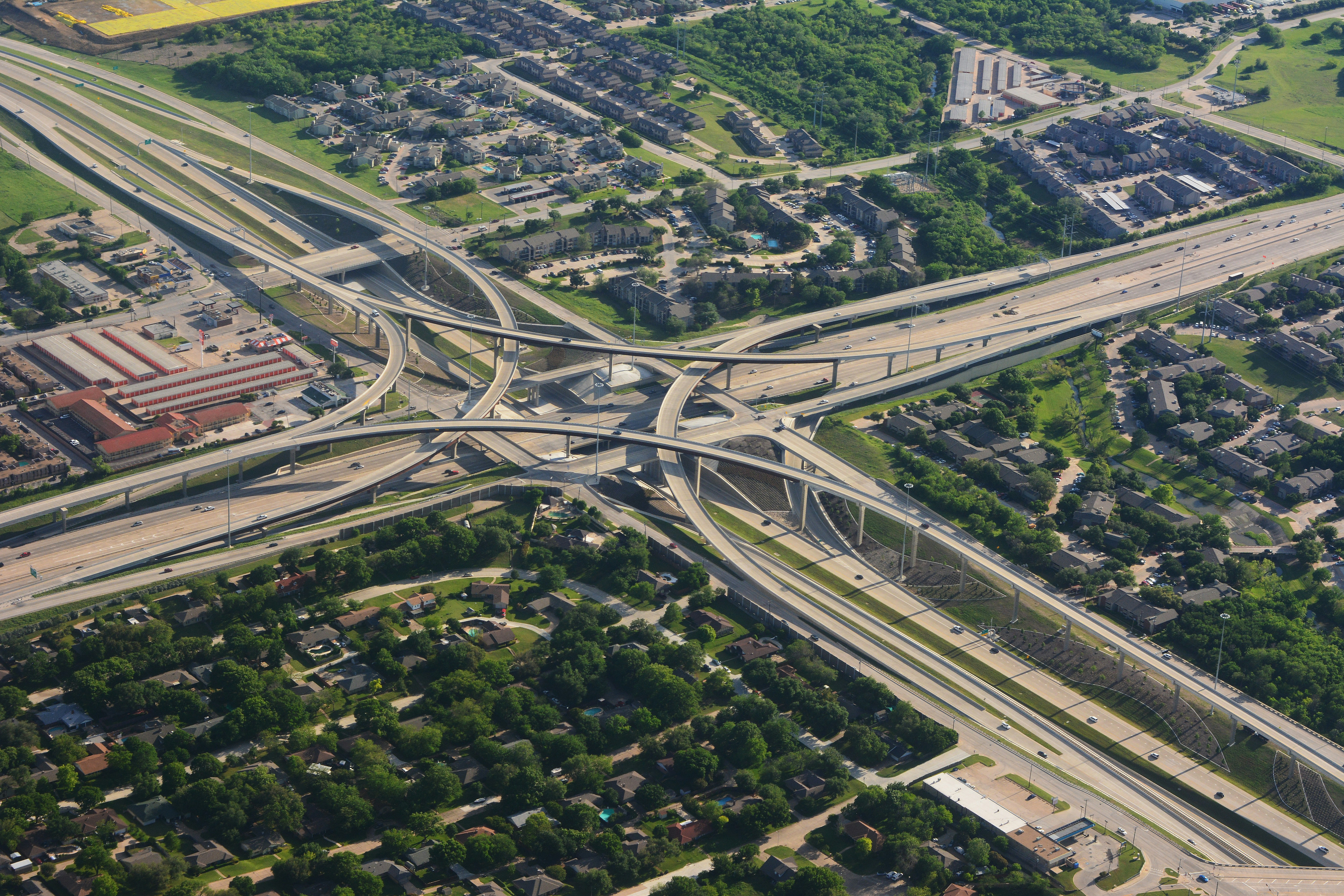# Train using multiple GPUs in fastai

Fastai makes training deep learning models on multiple GPUs a lot easier. In this blog, let's look at different approaches to train a model using multiple GPUs.In PyTorch, you can achieve Multi-GPU training using 2 different approaches.

• nn.DataParallel
• torch.distributed

nn.DataParallel is a good start for utilizing multiple GPUs parallelly. When you use it, you quickly realize that it does not use all the GPUs optimally. torch.distribued is more scalable and uses multiple GPUs in a more optimal fashion. In order to do this, you have to refactor your code and run the entire program as a script.

fastai framework makes this process a lot easier. A simple fastai pipeline has 3 major steps.

1. Data Pipeline - DataBlock.
2. Learner - Model, Metrics, Optimizers, DataLoaders.
3. Training loop - Baked with a lot of best practices.
dls = DataBlock(blocks=(ImageBlock, CategoryBlock),splitter=GrandparentSplitter(valid_name='val'),get_items=get_image_files, get_y=parent_label,item_tfms=[RandomResizedCrop(160), FlipItem(0.5)],batch_tfms=Normalize.from_stats(*imagenet_stats)).dataloaders(path, path=path, bs=64)learn = Learner(dls, xresnet50(n_out=10), metrics=[accuracy,top_k_accuracy]).to_fp16()learn.fit_flat_cos(2, 1e-3, cbs=MixUp(0.1))
Want to quickly try out this code?

I would assume that you are already familiar with this approach. The only change you need to make it work is to wrap the training loop with learn.distrib_ctx. So your training loop would look like this.

with learn.distrib_ctx():learn.fit_flat_cos(2, 1e-3, cbs=MixUp(0.1))

The one last important change we have to make while using a Jupyter notebook is to convert it as a script. Now we can launch the script can use multiple GPUs.

python -m fastai.launch fastai_multi_gpu.py

How about we try changing the model. timm is a popular repository making 100+ models available in PyTorch. So let's grab one of the most popular models and see how we can use it in our training loop. We have to only change the model that is passed to the learner and the rest remains the same.

timm_model = timm.create_model(model_name='tf_efficientnet_b4_ns',num_classes=10)learn = Learner(dls,timm_model, metrics=[accuracy,top_k_accuracy]).to_fp16()with learn.distrib_ctx():learn.fit_flat_cos(2, 1e-3, cbs=MixUp(0.1))

Now we can combine fastai and timm models to run on multi GPUs. Let's run few experiments on different numbers of GPUs and see how well the training scales.

EpochsGPUTime/secImg_size
1175256
1248256
1430256

All the results are without any optimization. The idea is to show we can quickly use multiple GPUs while using fastai framework. Using multi GPU can impact the model convergence in a positive or negative way. How it impacts depends on a lot of different factors like batch size, batch normalization, and image size.

Hope you enjoyed the post.

If you want to quickly try out this project or use the technique for your project then check out JarvisCloud - A simple and affordable GPU cloud platform.

Code for the blog is here. The main code is referenced from fastai example.

Train your Deep learning models on JarvisLabs.
Tags: